# Bank account CZ

We will deposit CZK 6,600 into the bank for 1 year with an interest rate of 2.5%. The interest tax is 15%. How many crowns will we receive after one year?

b =  6740.25 Kc

### Step-by-step explanation: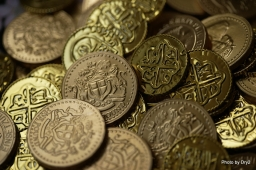Did you find an error or inaccuracy? Feel free to write us. Thank you!Tips to related online calculators

## Related math problems and questions:

• 3 years savingsSimple interest problem. Tereza has deposited CZK 100,000 in the bank with an annual interest rate of 1.5%. Interest is added each year and left aside. How many CZK (crowns) will have total after 3 years?
• Compound interestCompound interest: Clara deposited CZK 100,000 in the bank with an annual interest rate of 1.5%. Both money and interest remain deposited in the bank. How many CZK will be in the bank after 3 years?
• Deposit is pesosSally deposits Php.22,000 in her savings account. If the bank pays 1.5% interest per year, how much will she receive at the end of the year?
• Combined interest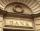Combined interest: Carol has deposited CZK 100,000 in the bank with an annual interest rate of 1.5%. The money was put into the account 5.5.2016 and withdraw them all before Christmas 20.12.2016. How much money did she withdraw?
• Interest p.a.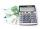Calculate the value of interest in period of 1 year from the deposit 62000 CZK at 8 percent interest rate, after deducting 15 percent tax.
• Bank deposit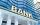Ms Jana after the withdraw amount of 2,500 euros after year saving in bank. What was her deposit if the interest rate was 2.5% p.a..
• BankPaul put 10000 in the bank for 6 years. Calculate how much you will have in the bank if he not pick earned interest or change deposit conditions. The annual interest rate is 3.5%, and the tax on interest is 10%.
• Profitable bank deposit 2012Calculate the value of what money lose creditor with a deposit € 9500 for 4 years if the entire duration is interest 2.6% p.a. and tax on interest is 19%. Annual inflation is 3.7% (Calculate what you will lose if you leave money lying idle at negative int
• Mr. Brown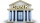Mr. Brown invested 15,000 euros in the bank for an annual term deposit. After the first year, he gained 600 euros. What was the annual interest rate of his deposit?
• DepositOh I total of \$15,000 deposited into simple interest accounts the annual simple interest rate on one account at 6% the annual simple interest rate on the second account at 7% how much should be invested in each account so that the total interest earned is
• Mr. Vojta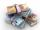Mr. Vojta put in the bank 35000 Kč. After a year bank, he credited with an interest rate of 2% of the deposit amount. How then will Mr. Vojta in the bank?
• Annual pensionCalculate the amount of money generating an annual pension of EUR 1000, payable at the end of the year and for a period of 10 years, shall be inserted into the bank to account with an annual interest rate of 2%
• The depositDeposit has been placed for one year at an annual rate of 4.5%. After the added interst amount rose to € 2,612.5. Determine initial deposit.
• Interst on savings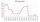The bank offers 1.6% interest. How many euros have to insert at the beginning if we received € 15 on the interest?
• DepositIf you deposit 719 euros at the beginning of each year, how much money we have at 1.3% (compound) interest after 9 years?
• Two accounts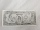Two accounts in the bank, one per year interest 2%, the second 3%. Total interest income 1900 USD. If interest rates were reversed, the yield would be USD 200 higher. What are the amounts on each account?
• Compound interest 4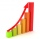3600 dollars is placed in an account with an annual interest rate of 9%. How much will be in the account after 25 years, to the nearest cent?